# Index Laws Worksheet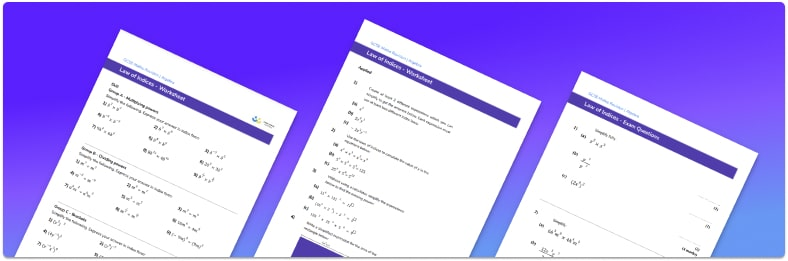Help your students prepare for their Maths GCSE with this free index laws worksheet of 53 questions and answers

• Section 1 of the index laws worksheet contains 45 skills-based index laws questions, in 3 groups to support differentiation
• Section 2 contains 4 applied index laws questions with a mix of worded problems and deeper problem solving questions
• Section 3 contains 4 foundation and higher level GCSE exam practice style questions involving all the laws of indices, either singularly or as a combination
• Answers and a mark scheme for all questions are provided
• Questions follow variation theory with plenty of opportunities for students to work independently at their own level
• All questions created by fully qualified expert secondary maths teachers
• Suitable for GCSE maths revision for AQA, OCR and Edexcel exam boards

• To receive this resource and regular emails with more free resources, blog posts and other Third Space updates, enter your email address and click below.

• This field is for validation purposes and should be left unchanged.

You can unsubscribe at any time (each email we send will contain an easy way to unsubscribe). To find out more about how we use your data, see our privacy policy.

### Index laws at a glance

The laws of indices are a set of rules which can be used to simplify questions involving indices. They are sometimes known as power laws or the rules of indices (exponents). A strong understanding of index form and index notation is helpful when using the laws of indices.

The first law of indices can be used when terms with the same base are multiplied together; in order to simplify the expression the powers can be added. The next law of indices can be used when terms with the same base are divided; in order to simplify the expression the powers can be subtracted. Another law of indices can be used when a term is raised to a power of a power; in order to simplify the expression the powers are multiplied.

Index laws also include negative indices (or negative powers) which involve writing the reciprocal of a number.

At Higher GCSE fractional indices are explored including square roots and cube roots. In the fractional indices the denominator of a fraction gives the root and the numerator gives the power the resulting term is raised to.

Looking forward, students can then progress to additional algebra worksheets, for example a simplifying expressions worksheet or simultaneous equations worksheet.For more teaching and learning support on Algebra our GCSE maths lessons provide step by step support for all GCSE maths concepts.

## Related worksheets

Brackets with Indices Worksheet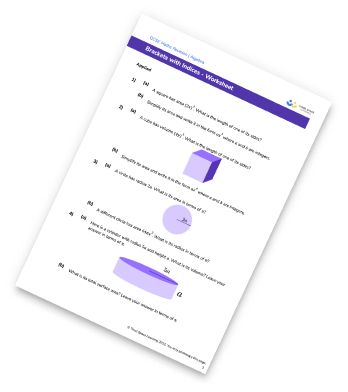Solving Quadratic Inequalities Worksheet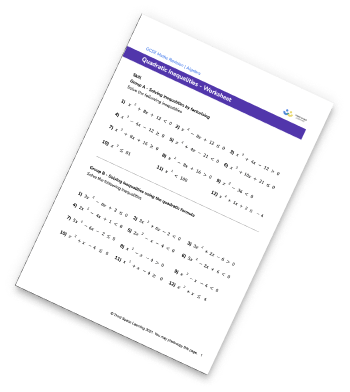Inequalities On A Number Line Worksheet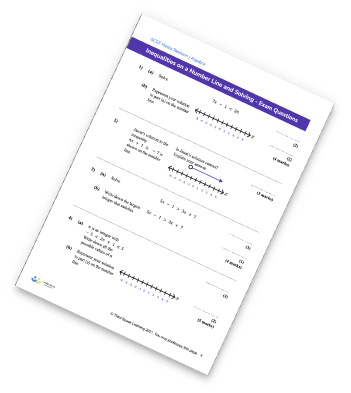Circle Graph Worksheet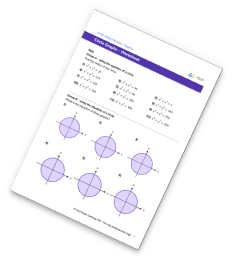## Do you have KS4 students who need more focused attention to succeed at GCSE?There will be students in your class who require individual attention to help them succeed in their maths GCSEs. In a class of 30, it’s not always easy to provide.

Help your students feel confident with exam-style questions and the strategies they’ll need to answer them correctly with our dedicated GCSE maths revision programme.

Lessons are selected to provide support where each student needs it most, and specially-trained GCSE maths tutors adapt the pitch and pace of each lesson. This ensures a personalised revision programme that raises grades and boosts confidence.

Find out more